Question

# Figure 1 shows a single robot joint/link driven through a gear ratio ? by an armature-controlled DC motor.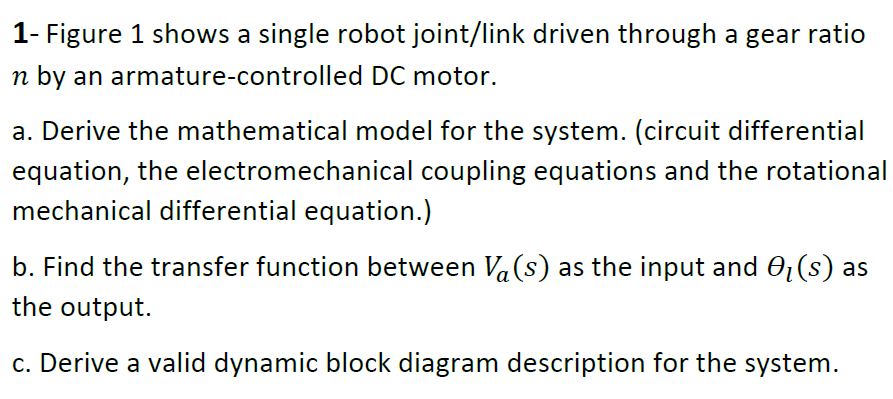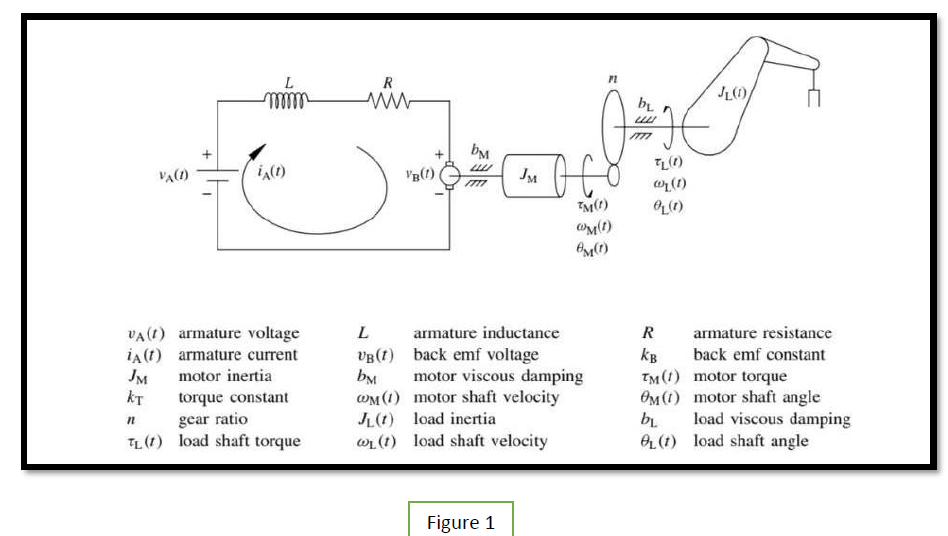We need at least 10 more requests to produce the answer.

0 / 10 have requested this problem solution

The more requests, the faster the answer.

All students who have requested the answer will be notified once they are available.

#### Earn Coins

Coins can be redeemed for fabulous gifts.

Similar Homework Help Questions
• ### An armature-controlled dc motor operates at steady state, with an armature drive voltage va = 10 ...

An armature-controlled dc motor operates at steady state, with an armature drive voltage va = 10 V. The motor runs at 600 rpm, and the armature current is found to be ia = 0.2 A. The armature resistance is Ra = 15 Ω. Determine: (a) The torque constant km of the motor (b) Electrical damping constant be (c) The efficiency under the given operating conditions, if the mechanical damping constant is bm = 8.25 × 10−5 N ⋅ m/rad/s (d)...

• ### Question 4 (Could be considered as Quiz 3- 4.5 pts). An armature-controlled DC motor of figure...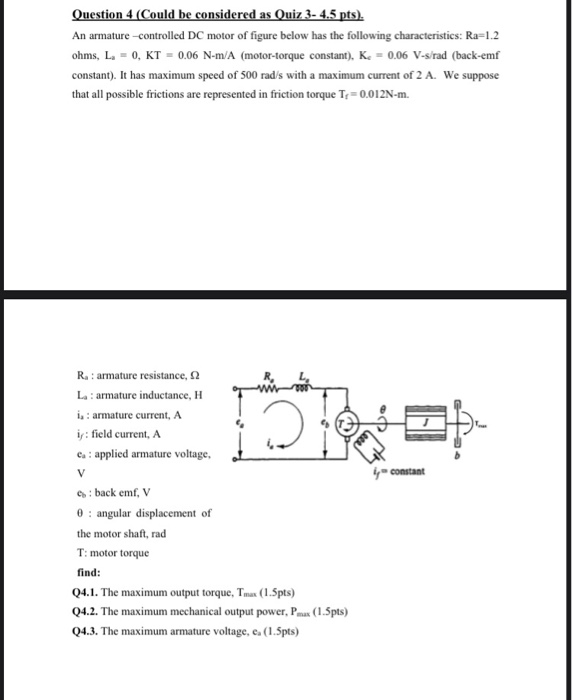Question 4 (Could be considered as Quiz 3- 4.5 pts). An armature-controlled DC motor of figure below has the following characteristics: Ra=1.2 ohms, L. - 0, KT = 0.06 N-m/A (motor-torque constant), K = 0.06 V-s/rad (back-emf constant). It has maximum speed of 500 rad's with a maximum current of 2 A. We suppose that all possible frictions are represented in friction torque Tr=0.012N-m. R: armature resistance, 2 La armature inductance, H is : armature current, A is: field current,...

• ### a single pdf file. Armature - Controlled DC Motor Representative Differential Equations di Ly da Ti...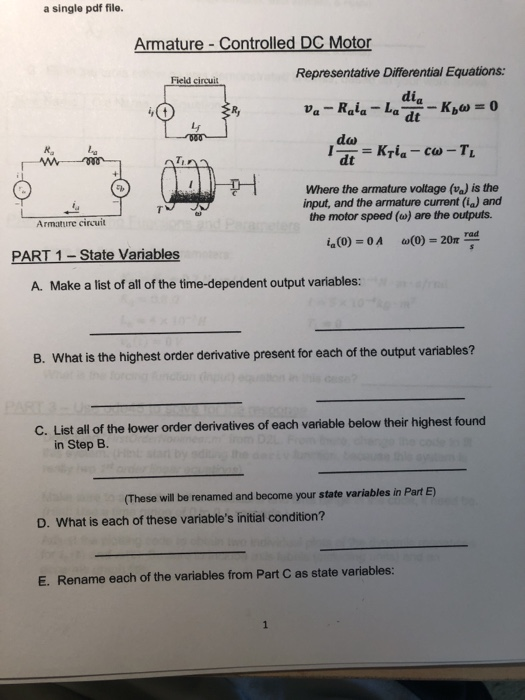a single pdf file. Armature - Controlled DC Motor Representative Differential Equations di Ly da Ti Where the armature voltage (%) is the input, and the armature current (a) and the motor speed (w) are the outputs. Armature circuit 4(0)-0 A a,(0) = 20π rad PART 1 -State Variables A. Make a list of all of the time-dependent output variables: B. What is the highest order derivative present for each of the output variables? C. List all of the lower...

• ### 3. The figure shows a DC servomotor system. Assume that the armature inductance of the DC...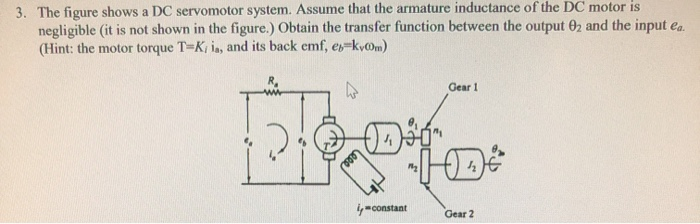3. The figure shows a DC servomotor system. Assume that the armature inductance of the DC motor is negligible (it is not shown in the figure.) Obtain the transfer function between the output 02 and the input ea. (Hint: the motor torque T-Kia, and its back emf, es kvm) Gear 1 y constant Gear 2

• ### Q5. (5 Marks) Suppose we have a one-link robot of L-1 m, controlled at the hinge by a motor. Ther...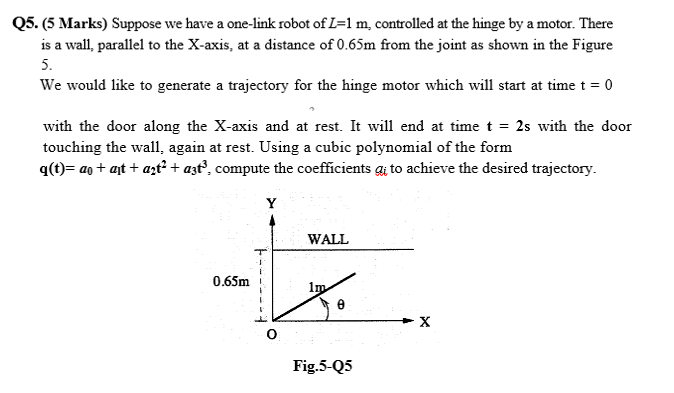Q5. (5 Marks) Suppose we have a one-link robot of L-1 m, controlled at the hinge by a motor. There is a wall, parallel to the X-axis, at a distance of 0.65m from the joint as shown in the Figure 5. We would like to generate a trajectory for the hinge motor which will start at time t 0 with the door along the X-axis and at rest. It will end at time t = 2s with the door q(t-...

• ### Robotics System subject Consider a single-link robot manipulator with a rotary joint. If initial angular position...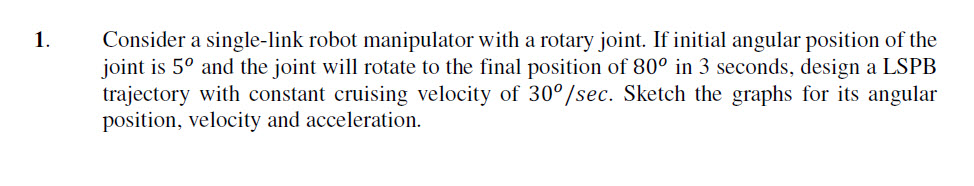Robotics System subject Consider a single-link robot manipulator with a rotary joint. If initial angular position of the joint is 5° and the joint will rotate to the final position of 80° in 3 seconds, design a LSPB trajectory with constant cruising velocity of 30°/sec. Sket position, velocity and acceleration I. ch the graphs for ts angular

• ### The field coil controlled DC motor is shown schematically in Figure Q2. (a) Define all the...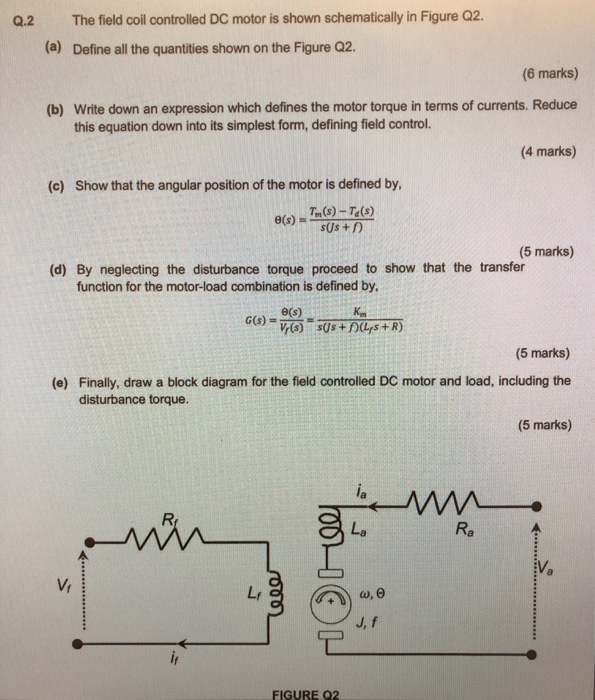The field coil controlled DC motor is shown schematically in Figure Q2. (a) Define all the quantities shown on the Figure Q2 Q.2 (6 marks) Write down an expression which defines the motor torque in terms of currents. Reduce 4 marks) (b) this equation down into its simplest form, defining field control. (c) Show that the angular position of the motor is defined by θ(s) = Tm(s)-Ta(s) (5 marks) (d) By neglecting the disturbance torque proceed to show that the...

• ### 3. A controlled single-phase fiull-wave controlled rectifier is connected in a de motor drive in Figure...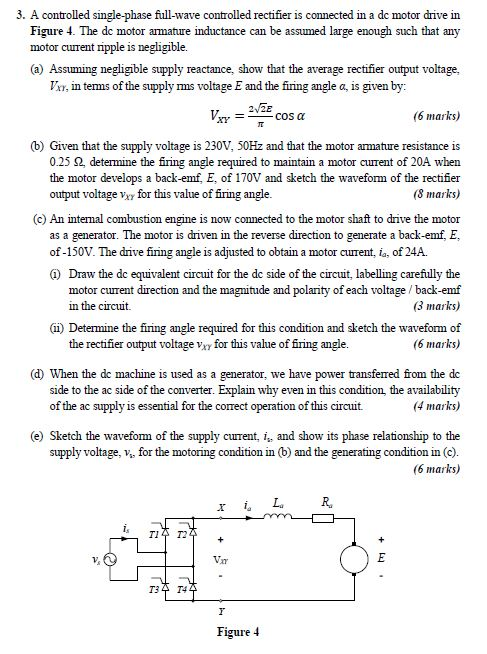3. A controlled single-phase fiull-wave controlled rectifier is connected in a de motor drive in Figure 4. The de motor armature inductance can be assumed large enough such that any motor curent ripple is negligible. (a) Assuming negligible supply reactance, show that the average rectifier output voltage V, in tems of the supply ms voltage E and the firing angle a, is given by 2/2E (6 marks) cos a _ (b) Given that the supply voltage is 230V, 50HZ and...

• ### 4. The figure below shows a simple control system for a robot joint. Find the step...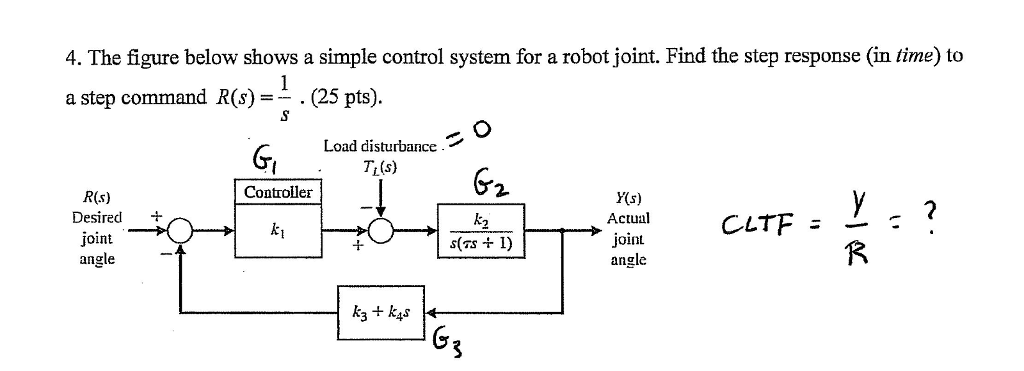4. The figure below shows a simple control system for a robot joint. Find the step response (in time) to a step command R (s)-. (25 pts). Load disturbance. I.T(s) Controller 2. R(s) Desired + joint angle Y(s) Acual joint angle I-

• ### The figure shows a gear-driven squeeze roll that is used to produce laminated wood product. The...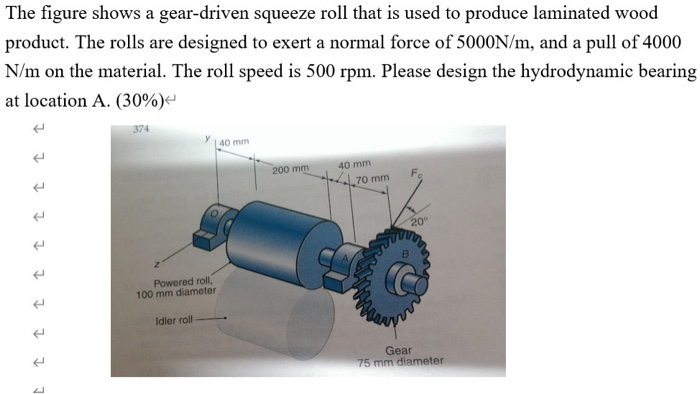The figure shows a gear-driven squeeze roll that is used to produce laminated wood product. The rolls are designed to exert a normal force of 5000N/m, and a pull of 4000 N/m on the material. The roll speed is 500 rpm. Please design the hydrodynamic bearing at location A. (30%) Į I 374 140 mm I 200 mm 40 mm L70 mm Į Î Î Î Powered roll, 100 mm diameter Î Idler roll- Î Gear 75 mm diameter E